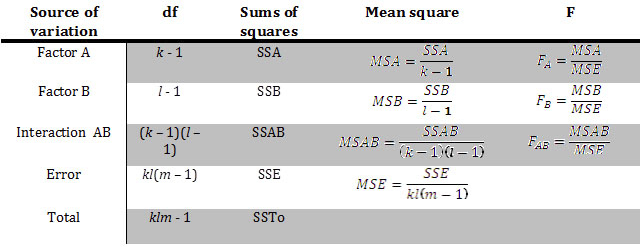top of page

# ANOVA / F-TestWe use the ANOVA (Analysis of Variance) aka F-Test when comparing the means of three or more groups.

One-Way ANOVA

A one-way ANOVA has one dependent variable and only one independent variable (or factor).

A one-way ANOVA has two hypotheses:

H (null): μ = μ = μ

H (alternative): at least one of the means are not equal.Example.

Two-Way ANOVA

A two-way ANOVA has one dependent variable and two independent variables (factors).

A two-way ANOVA has three null and alternative hypotheses:

H (null): No relationship between Dependent variable and Factor A

H (null): No relationship between Dependent variable and Factor A

H (null): No interaction between Factor A and Factor B

H (alternative): Relationship between Dependent variable and Factor A

H (alternative): Relationship between Dependent variable and Factor B

H (alternative): Interaction between Factor A and Factor BExamples

Calculators

The easiest way to calculate a z score, and find it's probability (p-value) is to use a statistics calculator.

Graphing calculators, statistical software programs (such as Excel, SPSS, R), or free online calculators are all able to Z-Tests, which give you the z-score and resulting probability for a value in a dataset.